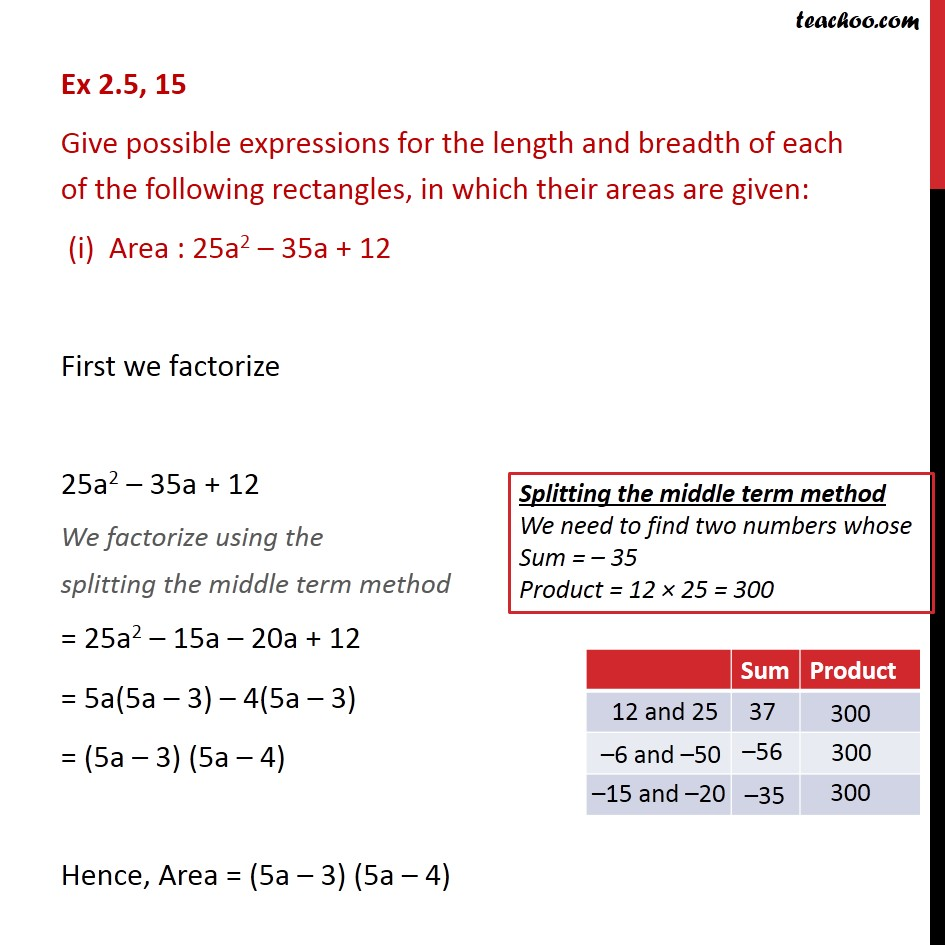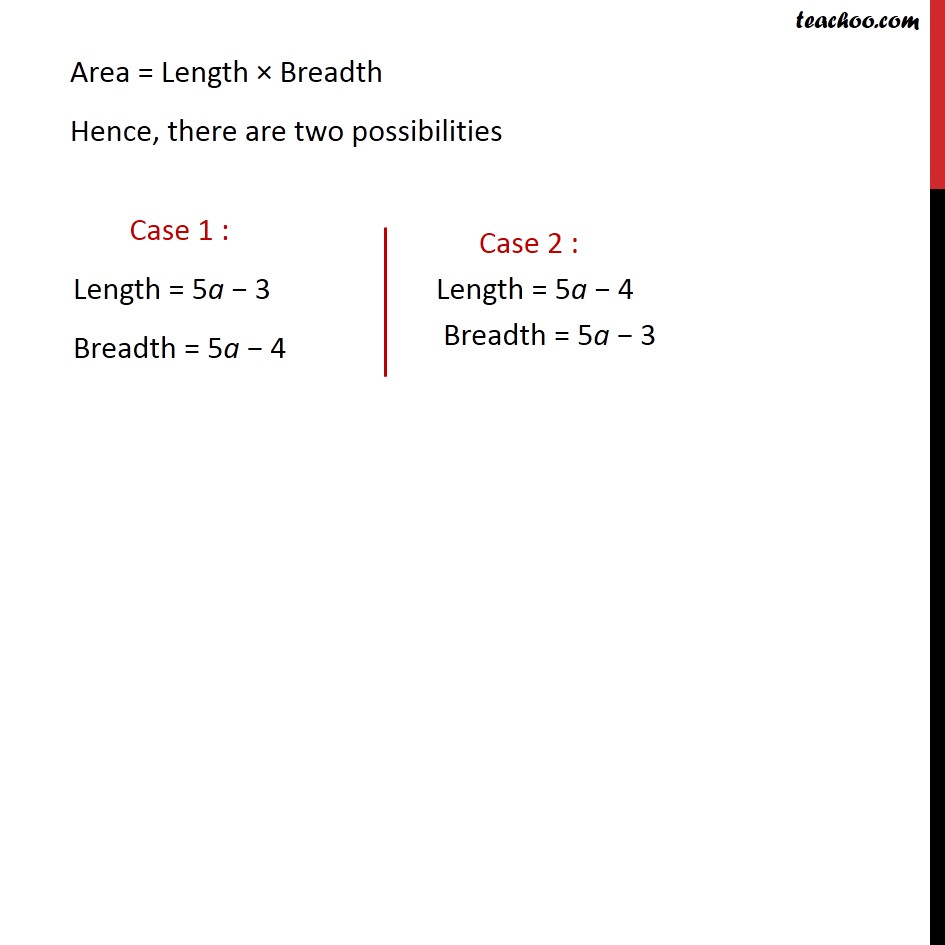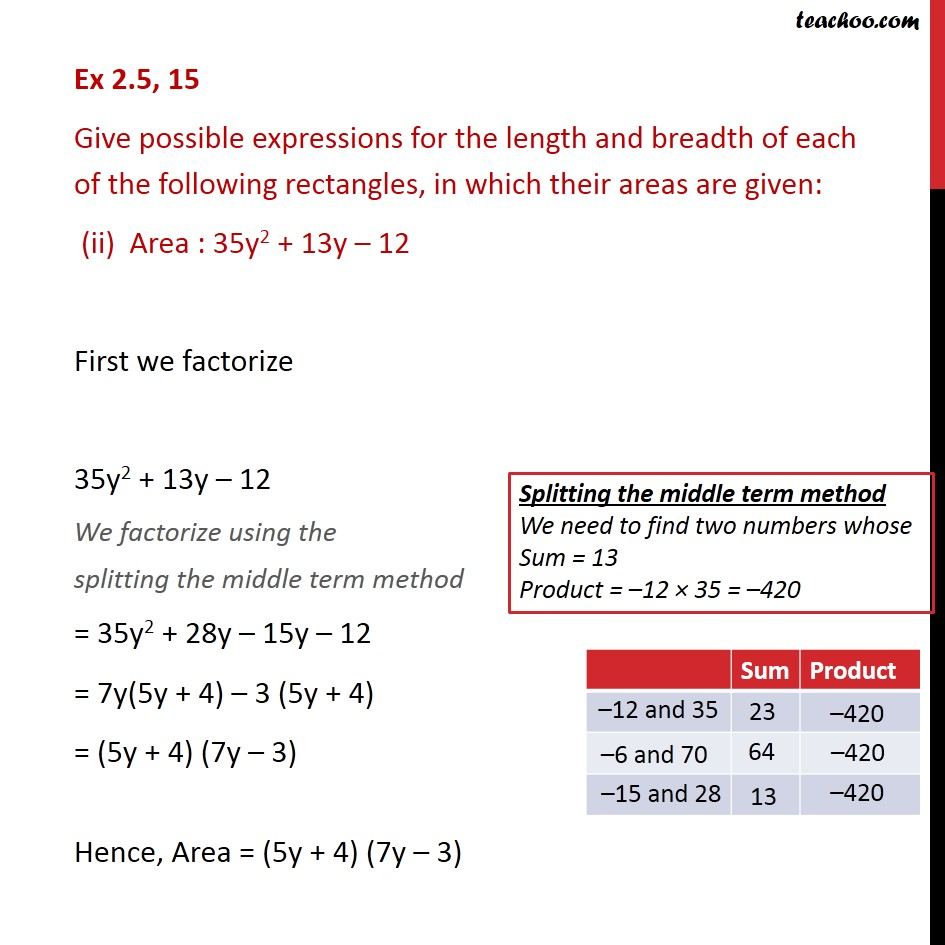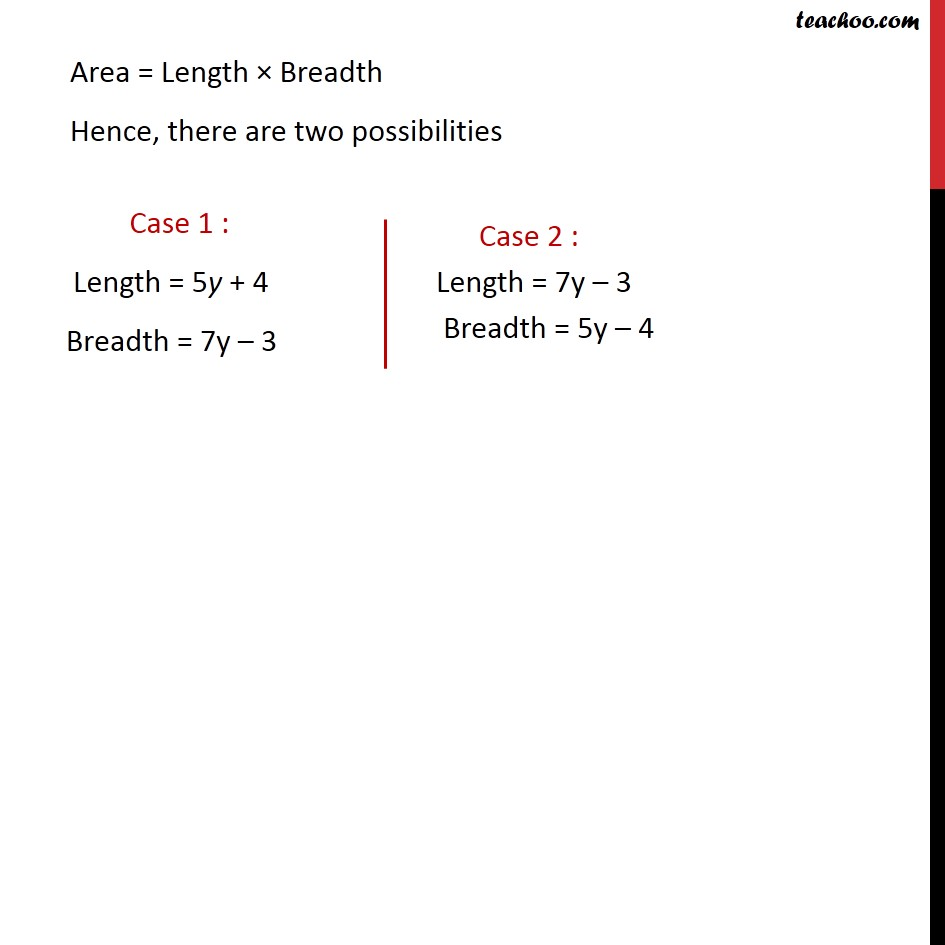Learn all Concepts of Polynomials Class 9 (with VIDEOS). Check - Polynomials Class 91. Chapter 2 Class 9 Polynomials
2. Serial order wise
3. Ex 2.5

Transcript

Ex 2.5, 15 Give possible expressions for the length and breadth of each of the following rectangles, in which their areas are given: (i) Area : 25a2 – 35a + 12 First we factorize 25a2 – 35a + 12 We factorize using the splitting the middle term method = 25a2 – 15a – 20a + 12 = 5a(5a – 3) – 4(5a – 3) = (5a – 3) (5a – 4) Hence, Area = (5a – 3) (5a – 4) Area = Length × Breadth Hence, there are two possibilities Ex 2.5, 15 Give possible expressions for the length and breadth of each of the following rectangles, in which their areas are given: (ii) Area : 35y2 + 13y – 12 First we factorize 35y2 + 13y – 12 We factorize using the splitting the middle term method = 35y2 + 28y – 15y – 12 = 7y(5y + 4) – 3 (5y + 4) = (5y + 4) (7y – 3) Hence, Area = (5y + 4) (7y – 3) Area = Length × Breadth Hence, there are two possibilities

Ex 2.5

Chapter 2 Class 9 Polynomials
Serial order wise